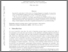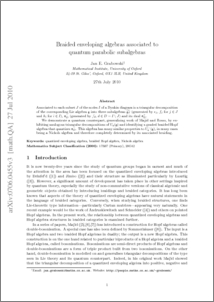# Braided enveloping algebras associated to quantum parabolic subalgebras

Grabowski, Jan (2011) Braided enveloping algebras associated to quantum parabolic subalgebras. Communications in Algebra, 39 (10). pp. 3491-3514. ISSN 0092-7872Preview
PDF (Braided enveloping algebras associated to quantum parabolic subalgebras)
0706_0455v3.pdf - Submitted Version

Associated to each subset $J$ of the nodes $I$ of a Dynkin diagram is a triangular decomposition of the corresponding Lie algebra $\mathfrak{g}$ into three subalgebras $\widetilde{\mathfrak{g}_{J}}$ (generated by $e_{j}$, $f_{j}$ for $j\in J$ and $h_{i}$ for $i\in I$), $\mathfrak{n}^{-}_{D}$ (generated by $f_{d}$, $d\in D=I\setminus J$) and its dual $\mathfrak{n}_{D}^{+}$. We demonstrate a quantum counterpart, generalising work of Majid and Rosso, by exhibiting analogous triangular decompositions of $U_{q}(\mathfrak{g})$ and identifying a graded braided Hopf algebra that quantizes $\mathfrak{n}_{D}^{-}$. This algebra has many similar properties to $U_{q}^{-}(\mathfrak{g})$, in many cases being a Nichols algebra and therefore completely determined by its associated braiding.View Item